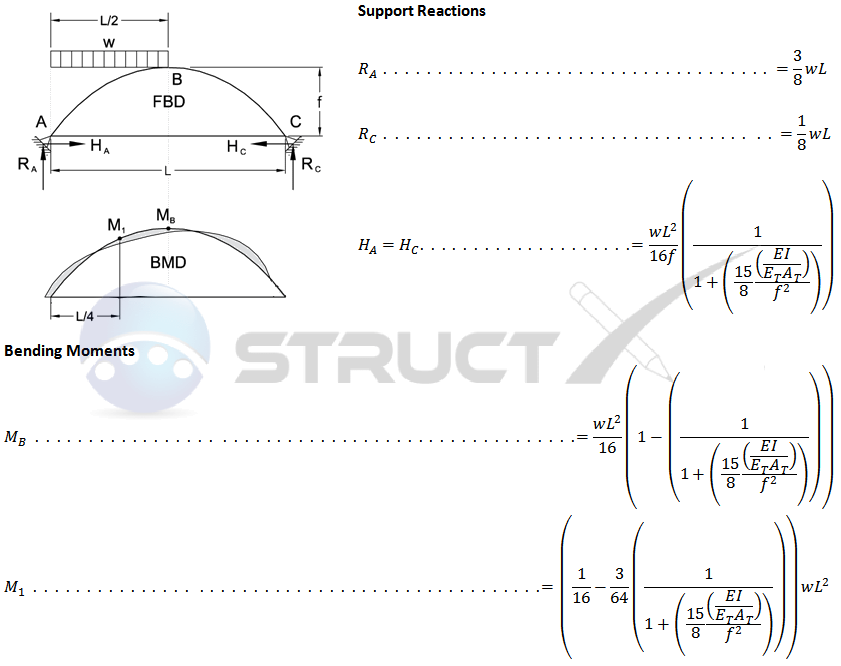# Two Hinge Tied Arch - Parabolic - Half UDLMore Arches# Notation and Units

## Metric and Imperial Units

The above arch formulas may be used with both imperial and metric units. As with all calculations care must be taken to keep consistent units throughout with examples of units which should be adopted listed below:

# Notation

• FBD = free body diagram
• BMD = bending moment diagram

• A, B & C = points of interest on arch
• AT = cross-sectional area of arch tie, in2 or mm2
• E = modulus of elasticity, psi or MPa
• ET = modulus of elasticity of arch tie, psi or MPa
• f = height of arch from supports, in or mm
• H = horizontal reaction load at bearing point, lbf or N
• I = second moment of area, in4 or mm4
• L = span length under consideration, in or mm
• M = maximum bending moment, lbf.in or Nmm
• NT = maximum tension load in arch tie, lbf.in or Nmm
• R = vertical reaction load at bearing point, lbf or N
• w = load per unit length, lbf/in or N/mm